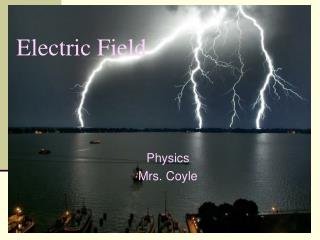Download PresentationElectric Field

# Electric Field - PowerPoint PPT PresentationDownload Presentation## Electric Field

- - - - - - - - - - - - - - - - - - - - - - - - - - - E N D - - - - - - - - - - - - - - - - - - - - - - - - - - -
##### Presentation Transcript

1. Electric Field Physics Mrs. Coyle

2. Analogy The electric field is the space around an electrical charge just like a gravitational field is the space around a mass.

3. Electric Field • Space around a charge.

4. What is the difference?

5. Van de Graaff Generator • Builds up static electric charges.

6. Electric Field Vector, E • E = F/ qo • qo , positive test charge • E is a vector quantity • Unit: N/C • E is analogous to the gravitational field, g, where g=F/m

7. Example 1 • A charge of 3µC is used to test the electric field of a central charge of 6C that causes a force of 800N. What is the magnitude of the electric field? • Answer: 2.7 x108 N/C

8. Electric Field http://higheredbcs.wiley.com/legacy/college/halliday/0471320005/simulations6e/index.htm?newwindow=true

9. Electric Field Lines

10. Electric Field Lines of two Positive Charges

11. Electric Field Lines • Lines that indicate the strength and direction of the electric field. • The more dense the lines, the stronger the field. • Electric field vectors are tangent to the curve. • Simulation http://online.cctt.org/physicslab/content/applets/pointcharges/elefi_z.htm

12. Conductors and Electric Fields (under electrostatic conditions) • “The electric field is zero inside a charged conductor”. • “Excess charge on an isolated conductor resides on the surface”. • “Excess charge accumulates on sharp points”. • Electric field lines meet the conductor perpendicular to the surface of the conductor.

13. Shielding • The electric field is zero inside a charged conductor.

14. Where are you safe during a thunderstorm? • In a car or • Outdoors

15. Which field is stronger? • A B

16. Electric Field for a Point Charge Using E=F/qo and Coulomb’s Law prove: E = k Q ______ r2 where Q is the central charge.

17. Example 2 • A test charge of +3µC is located 5m to the east of a -4µC charge. • A) Find the electric force felt by the test charge. • B) Find the electric field at that location. • Answer: 4.32x10-3 N, 1.44 x 103 N/C along the –x axis.

18. Example 3 • If a test charge is moved to a location three times as far as its original location, how does the electric field change?

19. Example 4 • Calculate the electric field felt by a test charge located half way between a charge of +1C and a charge of -3C, that are 2m apart. • Answer: 1.8 x 1010 N/C